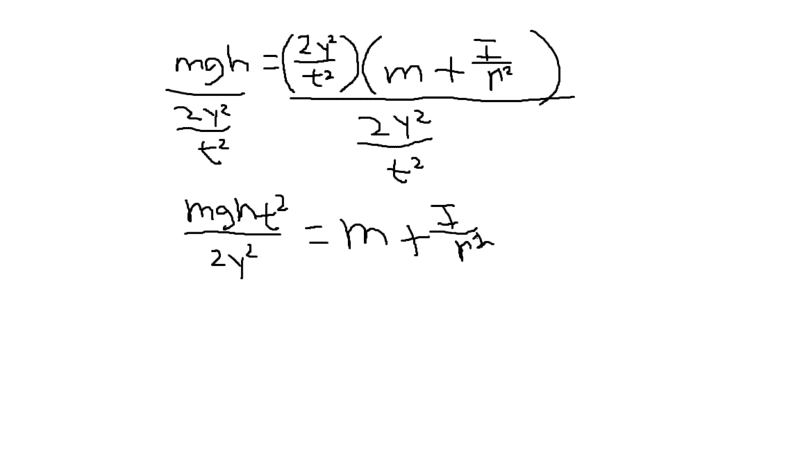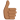# Solving For an Equation

## Homework Statement

I am trying to find I by rearranging the equation but i am having some trouble.

## Homework Equations

mgh=(2y^2/t^2)(m+I/r^2)

## The Attempt at a Solution

It is attachedbelow.

#### Attachments

dRic2
Gold Member
You're almost there!! Two more steps and it's done!

phinds
Gold Member
Well ... just keep going

so do i mutiply r^2 to the other side? then - the m?

dRic2
Gold Member
so do i mutiply r^2 to the other side? then - the m?

You can't multiply only one side of the equation

But you're close. BTW You have two possibilities

•HappyFlower
dRic2
Gold MemberPS: Try to learn LaTex if you need to write equations. You will save yourself the trouble of posting an image every time.